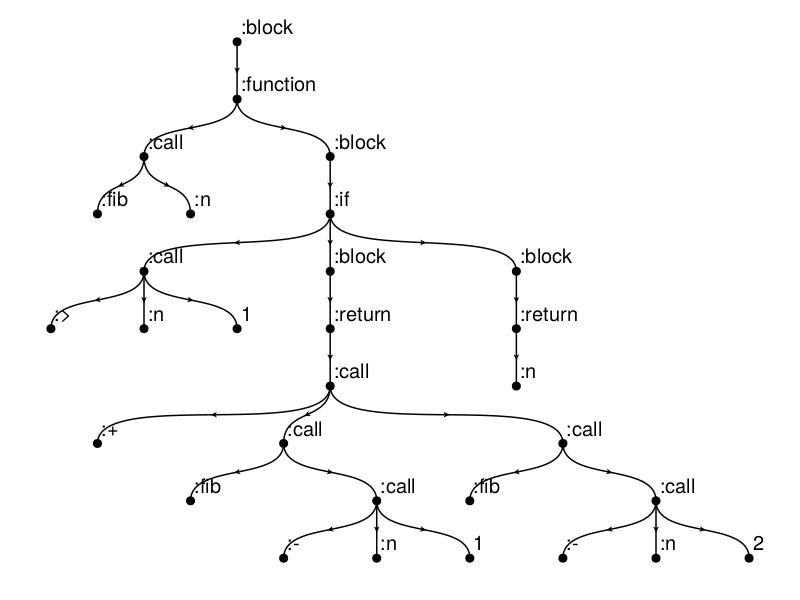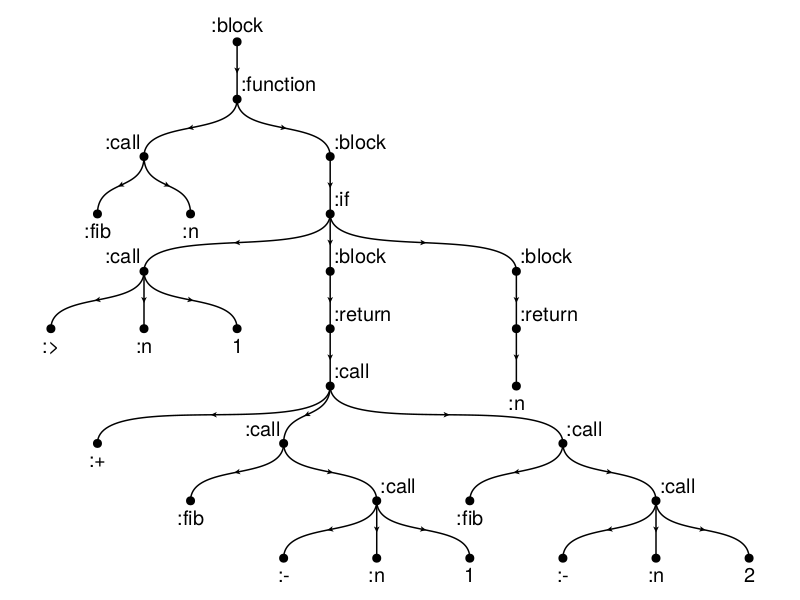# AST of a Julia function

In this example we are going to plot an abstract syntax tree of a Julia function using the Bucheim Layout from NetworkLayout.jl.

using CairoMakie
using Graphs
using GraphMakie
using NetworkLayout
using CairoMakie

The following code, which walks the AST and creats a SimpleDiGraph was taken and slightly modified from TreeView.jl. Thanks!

function walk_tree(ex; show_call=true)
g = SimpleDiGraph()
labels = Any[]
walk_tree!(g, labels, ex, show_call)
return (g, labels)
end

function walk_tree!(g, labels, ex, show_call)
top_vertex = vertices(g)[end]

if !(show_call) && ex.head == :call
f = ex.args   # the function name
push!(labels, f)
where_start = 2   # drop "call" from tree
else
end

for i in where_start:length(ex.args)
if isa(ex.args[i], Expr)
child = walk_tree!(g, labels, ex.args[i], show_call)
elseif !isa(ex.args[i], LineNumberNode)
n = vertices(g)[end]
push!(labels, ex.args[i])
end
end

end

The expression we want to look at is the recursive definition of the Fibonacci sequence.

expr = quote
function fib(n)
if n > 1
return fib(n-1) + fib(n-2)
else
return n
end
end
end

g, labels = walk_tree(expr, show_call=true)
nlabels_align = [(:left, :bottom) for v in vertices(g)]
fig, ax, p = graphplot(g; layout=Buchheim(),
nlabels=repr.(labels),
nlabels_distance=5,
nlabels_align,
tangents=((0,-1),(0,-1)))
hidedecorations!(ax); hidespines!(ax)This does not look nice yet! Lets tweak the align parameter of the nodes labels...

for v in vertices(g)
if isempty(inneighbors(g, v)) # root
nlabels_align[v] = (:center,:bottom)
elseif isempty(outneighbors(g, v)) #leaf
nlabels_align[v] = (:center,:top)
else
self = p[:node_pos][][v]
parent = p[:node_pos][][inneighbors(g, v)]
if self < parent # left branch
nlabels_align[v] = (:right,:bottom)
end
end
end
p.nlabels_align = nlabels_align